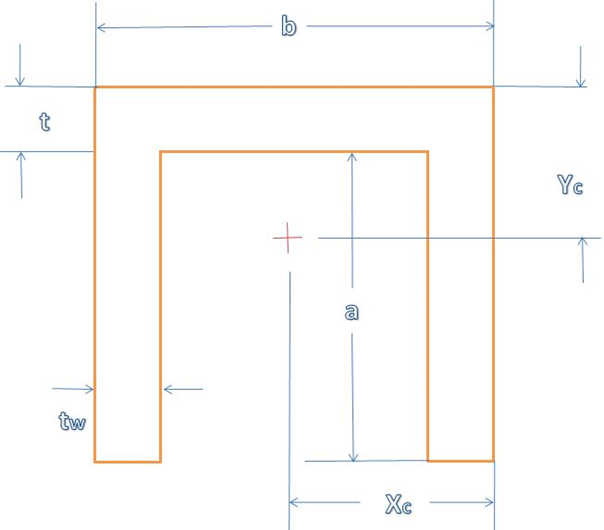# Section Properties of Channel Section

## Results

 Area {{Area:Decimal;0.00}} (length2) Distances from centroid to extremities (Xc) {{CentroidXc:Decimal;0.00}} (length) Distances from centroid to extremities (Yc) {{CentroidYc:Decimal;0.00}} (length) Moments and products of inertia (Ix) {{MomentofInertiaIx:Decimal;0.00}} (length4) Moments and products of inertia (Iy) {{MomentofInertiaIy:Decimal;0.00}} (length4) Radii of gyration about central axes (Rx) {{RadiiofGyrationRx:Decimal;0.00}} (length) Radii of gyration about central axes (Ry) {{RadiiofGyrationRy:Decimal;0.00}} (length) Plastic section moduli (Zx) {{PlasticZx:Decimal;0.00}} (length3) Plastic section moduli (Zy) {{PlasticZy:Decimal;0.00}} (length3) Shape factor (SFx) {{ShapeSFx:Decimal;0.00}} Shape factor (SFy) {{ShapeSFy:Decimal;0.00}}